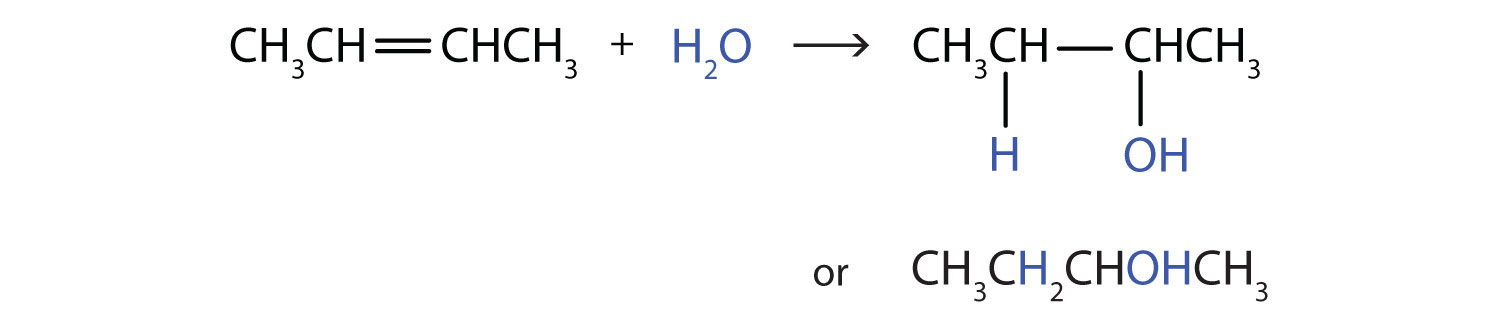# 13.4: Chemical Properties of Alkenes

Skills to Develop

• To write equations for the addition reactions of alkenes with hydrogen, halogens, and water

Alkenes are valued mainly for addition reactions, in which one of the bonds in the double bond is broken. Each of the carbon atoms in the bond can then attach another atom or group while remaining joined to each other by a single bond. Perhaps the simplest addition reaction ishydrogenation—a reaction with hydrogen (H2) in the presence of a catalyst such as nickel (Ni) or platinum (Pt).The product is an alkane having the same carbon skeleton as the alkene.

Alkenes also readily undergo halogenation—the addition of halogens. Indeed, the reaction with bromine (Br2) can be used to test for alkenes. Bromine solutions are brownish red. When we add a Br2 solution to an alkene, the color of the solution disappears because the alkene reacts with the bromine:Another important addition reaction is that between an alkene and water to form an alcohol. This reaction, called hydration, requires a catalyst—usually a strong acid, such as sulfuric acid (H2SO4):The hydration reaction is discussed later, where we deal with this reaction in the synthesis of alcohols.

Example $$\PageIndex{1}$$

Write the equation for the reaction between CH3CH=CHCH3 and each substance.

1. H2 (Ni catalyst)
2. Br2
3. H2O (H2SO4 catalyst)

SOLUTION

In each reaction, the reagent adds across the double bond.

1.2.3.Exercise $$\PageIndex{1}$$

Write the equation for each reaction.

1. CH3CH2CH=CH2 with H2 (Ni catalyst)
2. CH3CH=CH2 with Cl2
3. CH3CH2CH=CHCH2CH3 with H2O (H2SO4 catalyst)

## Concept Review Exercises

1. What is the principal difference in properties between alkenes and alkanes? How are they alike?
2. If C12H24 reacts with HBr in an addition reaction, what is the molecular formula of the product?

1. Alkenes undergo addition reactions; alkanes do not. Both burn.
2. C12H24Br2

## Key Takeaway

• Alkenes undergo addition reactions, adding such substances as hydrogen, bromine, and water across the carbon-to-carbon double bond.

## Exercises

1. Complete each equation.

1. (CH3) 2C=CH2 + Br2
2. $$\mathrm{CH_2\textrm{=C}(CH_3)CH_2CH_3 + H_2 \xrightarrow{Ni}}$$
3.2. Complete each equation.

1. $$\mathrm{CH_2\textrm{=CHCH=C}H_2 + 2H_2\xrightarrow{Ni}}$$
2. $$\mathrm{(CH_3)_2\textrm{C=C}(CH_3)_2 + H_2O \xrightarrow{H_2SO_4}}$$
3.3.